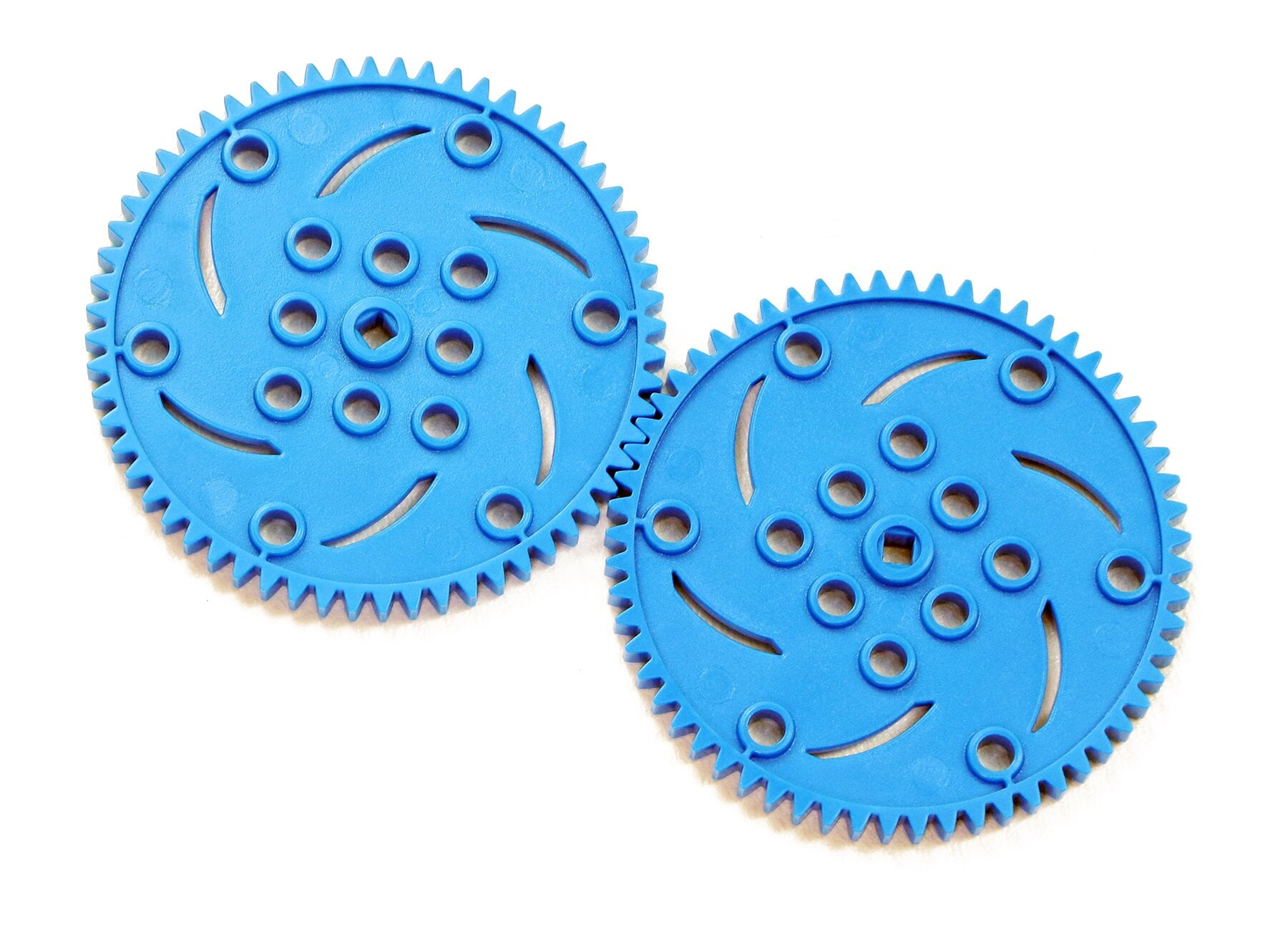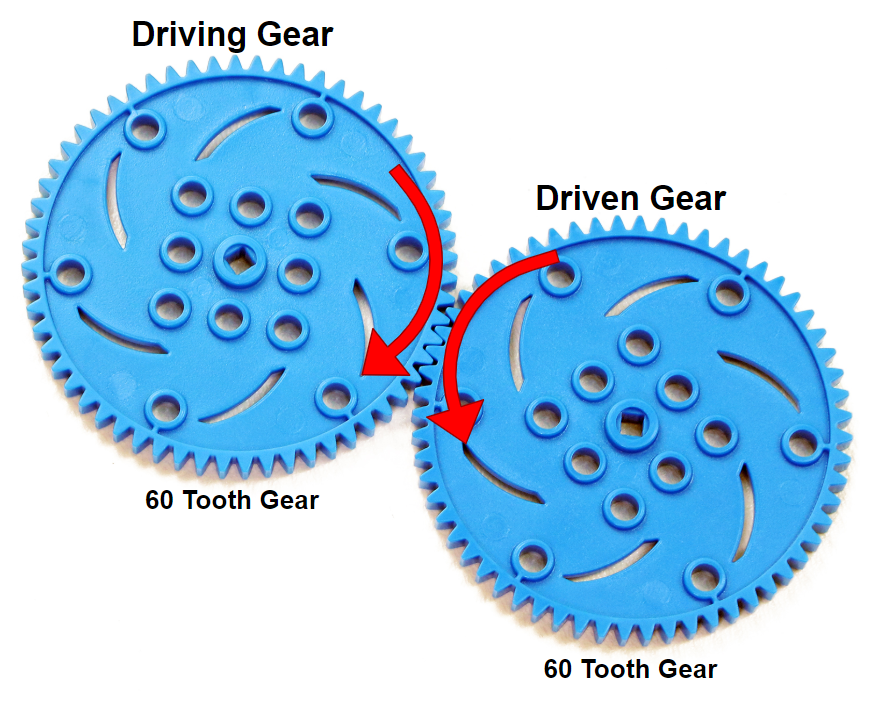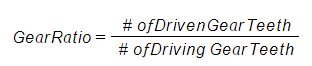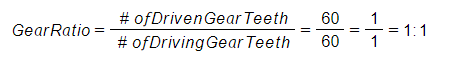STEMLabs IQ
Lab 12 - M.A.D. Box### Gears

Gears look like disks with teeth around their edges. It is important to notice that their teeth are equally spaced because gears work by having their teeth meshed together, as shown in the image above. When one gear turns, it turns the next one because their teeth are positioned between each other, which is known as being meshed.

Gears are typically mounted, or connected to other parts, by a shaft or base. So gears are used to transmit rotary motion, or power, from one shaft to another. The shaft is usually positioned at the gear's center. In the image above of the VEX IQ Gears, the center hole to pass a shaft through is the square one because the IQ Shafts are square.

One of the main ways to define a gear is by the number of teeth that it has.

### Meshed Gears

When two gears are meshed together, one gear turns the next. The gear that is doing the turning first is called the driving gear. The driving gear can be thought of as a type of input. The gear that is being turned by the first gear is called the driven gear. The driven gear is therefore the output.

Watch the animation below to see meshed gears in action.

Video file

You should have noticed that the driving gear and driven gear turn in opposite directions. They have to spin in opposite directions because their teeth are meshed and they rotate at their centers.

### Gear RatiosA gear ratio is a comparison of the input (driving gear) to output (driven gear) and is calculated by considering each meshed gear's number of teeth.

In the example above, the driving gear (input) and the driven gear (output) both have 60 teeth.

Here is the formula for calculating a gear ratio:Let's use the example of the two 60 Tooth Gears above because it's a simple ratio to calculate.The gear ratio of these two meshed gears is 1:1 which means each time the driving gear (input) turns one full rotation, the driven gear (output) also turns one full rotation.